# Localization at a prime ideal

Let$A$ be a commutative unital ring and$P$ a prime ideal in$A$. Then, the localization of$A$ at$P$ is defined as follows:
• As a set, it is the collection of fractions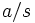$a/s$ where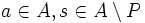$a \in A, s \in A \setminus P$, subject to the equivalence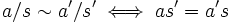$a/s \sim a'/s' \iff as' = a's$.
• The operations are defined as follows:$a/s + a'/s' = (as' + a's)/(ss')$ and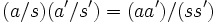$(a/s) (a'/s') = (aa')/(ss')$$A$ embeds naturally as a subset of$A_P$. If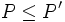$P \le P'$ are prime ideals in$A$, we have an embedding from$A_{P'}$ into$A_P$. In fact, if$A$ is an [[integral domain], then all the$A_P$s are contained inside the residue field of$A$. Further, their intersection is exactly$A$.